# Newspaper scraping using Python and News API

There are mainly two ways to extract data from a website:

• Use the API of the website (if it exists). For example, Facebook has the Facebook Graph API which allows retrieval of data posted on Facebook.
• Access the HTML of the webpage and extract useful information/data from it. This technique is called web scraping or web harvesting or web data extraction.

In this article, we will be using the API of `newsapi`. You can create your own API key by clicking here.

Examples: Let’s determine the concern of a personality like states president cited by newspapers, let’s take the case of MERKEL

 `import` `pprint ` `import` `requests ` ` `  ` `  `secret ``=` `"Your API"` `  `  `# Define the endpoint ` `url ``=` `'https://newsapi.org/v2/everything?'` `  `  `# Specify the query and ` `# number of returns ` `parameters ``=` `{ ` `    ``'q'``: ``'merkel'``, ``# query phrase ` `    ``'pageSize'``: ``100``,  ``# maximum is 100 ` `    ``'apiKey'``: secret ``# your own API key ` `} ` `  `  `# Make the request ` `response ``=` `requests.get(url,  ` `                        ``params ``=` `parameters) ` `  `  `# Convert the response to  ` `# JSON format and pretty print it ` `response_json ``=` `response.json() ` `pprint.pprint(response_json) `

Output: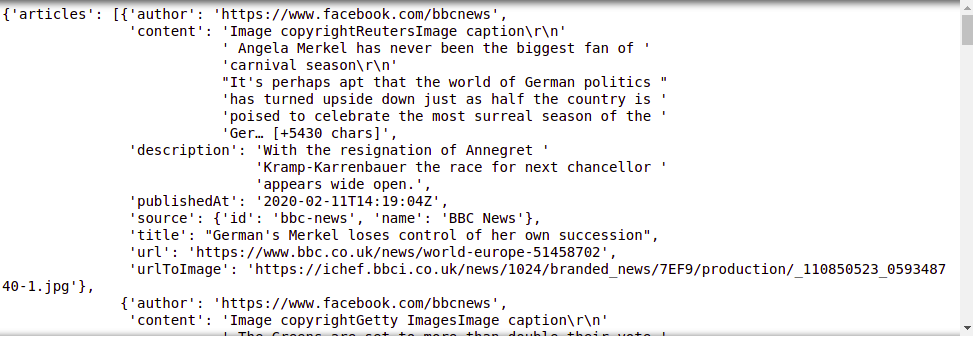Let’s combine all texts and sort the words from the greatest number to lower.

 `from` `wordcloud ``import` `WordCloud ` `import` `matplotlib.pyplot as plt ` ` `  ` `  `text_combined ``=` `'' ` ` `  `for` `i ``in` `response_json[``'articles'``]: ` `     `  `    ``if` `i[``'description'``] !``=` `None``: ` `        ``text_combined ``+``=` `i[``'description'``] ``+` `' '` `         `  `wordcount``=``{} ` `for` `word ``in` `text_combined.split(): ` `    ``if` `word ``not` `in` `wordcount: ` `        ``wordcount[word] ``=` `1` `    ``else``: ` `        ``wordcount[word] ``+``=` `1` ` `  `for` `k,v, ``in` `sorted``(wordcount.items(), ` `                   ``key``=``lambda` `words: words[``1``],  ` `                   ``reverse ``=` `True``): ` `    ``print``(k,v) `

Output: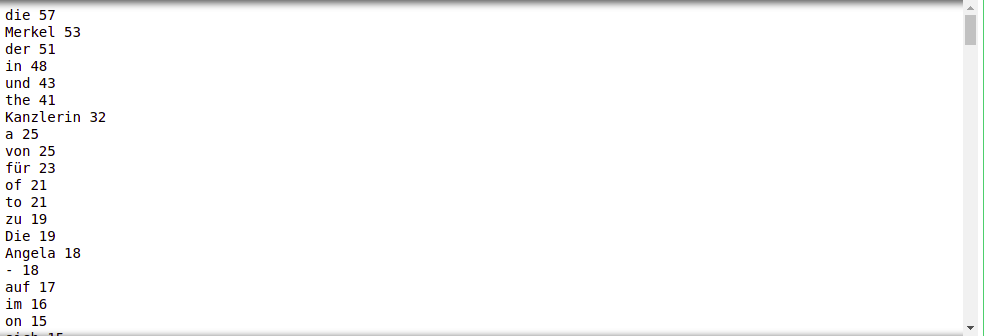This evaluation is ambiguous, we can make it more clear if we delete bad or usless words. Let’s define some of bad_words shown below

bad_words = [“a”, “the”, “of”, “in”, “to”, “and”, “on”, “de”, “with”,
“by”, “at”, “dans”, “ont”, “été”, “les”, “des”, “au”, “et”,
“après”, “avec”, “qui”, “par”, “leurs”, “ils”, “a”, “pour”,
“les”, “on”, “as”, “france”, “eux”, “où”, “son”, “le”, “la”,
“en”, “with”, “is”, “has”, “for”, “that”, “an”, “but”, “be”,
“are”, “du”, “it”, “à”, “had”, “ist”, “Der”, “um”, “zu”, “den”,
“der”, “-“, “und”, “für”, “Die”, “von”, “als”,
“sich”, “nicht”, “nach”, “auch” ]

Now we can delete and format the text by deleting bad words

 `# initializing bad_chars_list  ` `bad_words ``=` `[``"a"``, ``"the"` `, ``"of"``, ``"in"``, ``"to"``, ``"and"``, ``"on"``, ``"de"``, ``"with"``,  ` `             ``"by"``, ``"at"``, ``"dans"``, ``"ont"``, ``"été"``, ``"les"``, ``"des"``, ``"au"``, ``"et"``,  ` `             ``"après"``, ``"avec"``, ``"qui"``, ``"par"``, ``"leurs"``, ``"ils"``, ``"a"``, ``"pour"``,  ` `             ``"les"``, ``"on"``, ``"as"``, ``"france"``, ``"eux"``, ``"où"``, ``"son"``, ``"le"``, ``"la"``, ` `             ``"en"``, ``"with"``, ``"is"``, ``"has"``, ``"for"``, ``"that"``, ``"an"``, ``"but"``, ``"be"``,  ` `             ``"are"``, ``"du"``, ``"it"``, ``"à"``, ``"had"``, ``"ist"``, ``"Der"``, ``"um"``, ``"zu"``, ``"den"``,  ` `             ``"der"``, ``"-"``, ``"und"``, ``"für"``, ``"Die"``, ``"von"``, ``"als"``, ` `             ``"sich"``, ``"nicht"``, ``"nach"``, ``"auch"`  `]  ` ` `  ` `  `r ``=` `text_combined.replace(``'\s+'``, ` `                          ``' '``).replace(``','``,  ` `                                       ``' '``).replace(``'.'``, ` `                                                    ``' '``) ` `words ``=` `r.split() ` `rst ``=` `[word ``for` `word ``in` `words ``if`  `       ``( word.lower() ``not` `in` `bad_words  ` `        ``and` `len``(word) > ``3``) ] ` ` `  `rst ``=` `' '``.join(rst) ` `  `  `wordcount``=``{} ` ` `  `for` `word ``in` `rst.split(): ` `     `  `    ``if` `word ``not` `in` `wordcount: ` `        ``wordcount[word] ``=` `1` `    ``else``: ` `        ``wordcount[word] ``+``=` `1` `  `  `for` `k,v, ``in` `sorted``(wordcount.items(), ` `                   ``key``=``lambda` `words: words[``1``], ` `                   ``reverse ``=` `True``): ` `    ``print``(k,v) `

Output: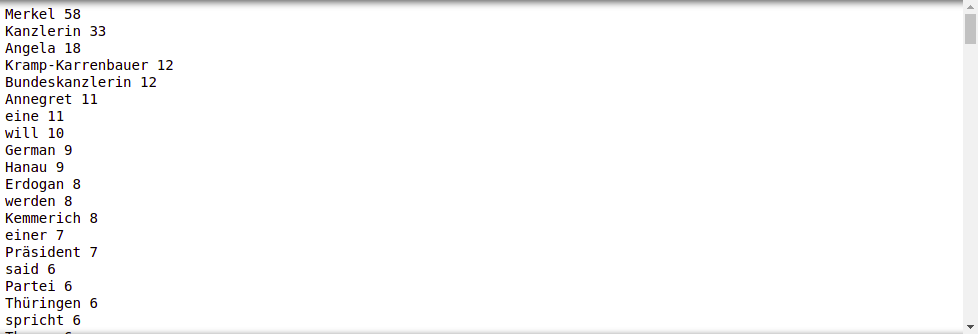Let’s plot the output

 `word ``=` `WordCloud(max_font_size ``=` `40``).generate(rst) ` `plt.figure() ` `plt.imshow(word, interpolation ``=``"bilinear"``) ` `plt.axis(``"off"``) ` `plt.show() `

Output: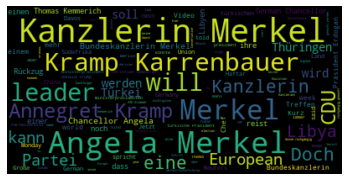As you see in the descriptions of articles, the most dominant concern with Merkel is his defense minister Kramp-Karrenbauer, Kanzlerin just means female chancellor. We can do the same work using titles only

 `title_combined ``=` `'' ` ` `  `for` `i ``in` `response_json[``'articles'``]: ` `    ``title_combined ``+``=` `i[``'title'``] ``+` `' '` `     `  `titles ``=` `title_combined.replace(``'\s+'``, ` `                                ``' '``).replace(``','``, ` `                                             ``' '``).replace(``'.'``, ` `                                                          ``' '``) ` `words_t ``=` `titles.split() ` `result ``=` `[word ``for` `word ``in` `words_t ``if` `          ``( word.lower() ``not` `in` `bad_words ``and` `           ``len``(word) > ``3``) ] ` ` `  `result ``=` `' '``.join(result) ` `  `  `wordcount``=``{} ` ` `  `for` `word ``in` `result.split(): ` `     `  `    ``if` `word ``not` `in` `wordcount: ` `        ``wordcount[word] ``=` `1` `    ``else``: ` `        ``wordcount[word] ``+``=` `1` ` `  `word ``=` `WordCloud(max_font_size``=``40``).generate(result) ` `plt.figure() ` `plt.imshow(word, interpolation``=``"bilinear"``) ` `plt.axis(``"off"``) ` `plt.show() `

Output: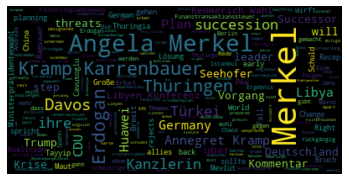From titles, we found out that the most concern with Merkel is Ardogan, turkey president.

My Personal Notes arrow_drop_upCheck out this Author's contributed articles.

If you like GeeksforGeeks and would like to contribute, you can also write an article using contribute.geeksforgeeks.org or mail your article to contribute@geeksforgeeks.org. See your article appearing on the GeeksforGeeks main page and help other Geeks.

Please Improve this article if you find anything incorrect by clicking on the "Improve Article" button below.

Article Tags :

1

Please write to us at contribute@geeksforgeeks.org to report any issue with the above content.